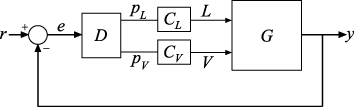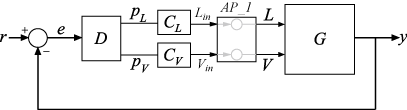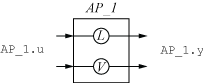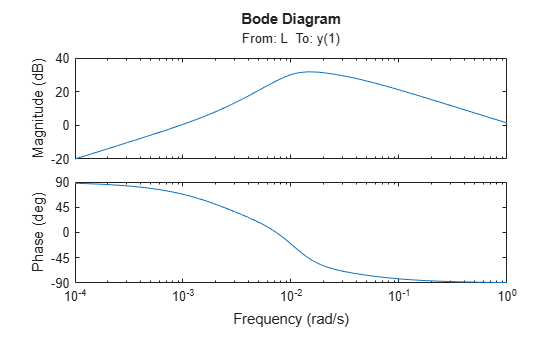Documentation

## Control System with Multichannel Analysis Points

This example shows how to insert multichannel analysis points in a generalized state-space model of a MIMO control system.

Consider the following two-input, two-output control system.The plant `G` has two inputs and two outputs. Therefore, the line marked `y` in the block diagram represents two signals, `y(1)` and `y(2)`. Similarly, `r` and `e` each represent two signals.

Suppose you want to create tuning requirements or extract responses that require injecting or measuring signals at the locations `L` and `V`. To do so, create an `AnalysisPoint` block and include it in the closed-loop model of the control system as shown in the following illustration.To create a model of this system, first create the numeric LTI models and control design blocks that represent the plant and controller elements. `D` is a tunable gain block, and `C_L` and `C_V` are tunable PI controllers. Suppose the plant model is the following:

`$G\left(s\right)=\frac{1}{75s+1}\left[\begin{array}{cc}87.8& -86.4\\ 108.2& -109.6\end{array}\right].$`

```s = tf('s'); G = [87.8 -86.4 ; 108.2 -109.6]/(75*s+1); D = tunableGain('Decoupler',eye(2)); C_L = tunablePID('C_L','pi'); C_V = tunablePID('C_V','pi');```

Create an `AnalysisPoint` block that bundles together the `L` and `V` channels.

`AP_1 = AnalysisPoint('AP_1',2)`
```AP_1 = Multi-channel analysis point at locations: AP_1(1) AP_1(2) Type "ss(AP_1)" to see the current value and "get(AP_1)" to see all properties. ```

For convenience, rename the channels to match the corresponding signals.

`AP_1.Location = {'L';'V'}`
```AP_1 = Multi-channel analysis point at locations: L V Type "ss(AP_1)" to see the current value and "get(AP_1)" to see all properties. ```

The following diagram illustrates the input names, output names, and channel names (locations) in the block `AP_1`.The input and output names of the `AnalysisPoint` block are distinct from the channel names. Use the channel names to refer to the analysis-point locations when extracting responses or defining design goals for tuning. You can use the input and output names `AP_1.u` and `AP_1.y`, for example, when interconnecting blocks using the `connect` command.

You can now build the closed-loop model of the control system. First, join all the plant and controller blocks along with the first `AnalysisPoint` block.

`GC = G*AP_1*append(C_L,C_V)*D;`

Then, close the feedback loop. Recall that `GC` has two inputs and outputs.

`CL = feedback(GC,eye(2));`

You can now use the analysis points for analysis or tuning. For example, extract the SISO closed-loop transfer function from `'L'` to the first output. Assign a name to the output so you can reference it in analysis functions. The software automatically expands the assigned name `'y'` to the vector-valued output signals `{y(1),y(2)}`.

```CL.OutputName = 'y'; TLy1 = getIOTransfer(CL,'L','y(1)'); bodeplot(TLy1);```